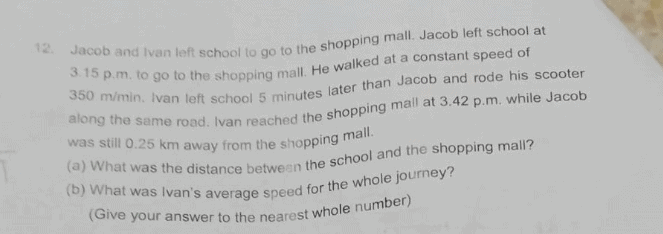# QuestionNeeds assistance to solve these time questions.
Thank you.

Thank you for the solutions for this questions

0 Replies 1 Like ✔Accepted Answer

For Jacob,

Time elapsed = 27 mins

Distance travelled = 350 m/min x 27 mins = 9450 m

(a) Total Distance between school and shopping mall = 9450 m + 250 m = 9700 m

For Ivan,

Time elapsed = 27 mins – 5 mins = 22 mins

(b) Average speed = 9700 m / 22 mins = 441 m/min (to the nearest whole number)